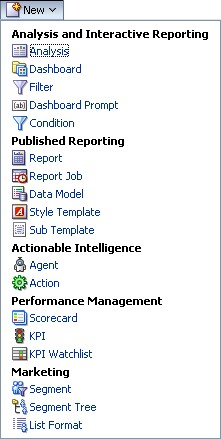# OBIEE - How to pivot a character column ?

The pivot of a character is just an easy way to split one character column in two or more columns.

By default, the pivot view take the measures (the column set with an aggregation rule) and place them in the intersection of the X and Y axis. You will then never find a column without an aggregation rule that contains a character data type.

## Solution

To overcome this issue, you have to:

• set an aggregation rule such as max, min in your pivot view
• or set on the character column this formula :
````min(Table.CharacterColumn by Table.XAxisColumn, Table.YAxisColumn)`
```

where XAxisColumn is the row and YAxisColumn is the column

## Supplement

### How to show a table of three columns ?

Replace V_NumberOfColumn by the number of column.

The Number of column must be a double and not an integer. Integer = 2, Double = 2.0 in C, C++

X Axis :

````MOD(RCOUNT(Table.CharacterColumn),V_NumberOfColumn)`
```

Y Axis :

````FLOOR(RCOUNT(Table.CharacterColumn) / V_NumberOfColumn)`
```

For the table :

``````min(Table.Column by MOD(RCOUNT(Table.CharacterColumn),V_NumberOfColumn),
FLOOR(RCOUNT(Table.CharacterColumn) / V_NumberOfColumn))```
```

### How to show an URL of an image ?

You can then set the column format as an HTML and modify the column format to add the HTML nodes as :

``````'<a href="' || min(Table.CharacterColumn by MOD(RCOUNT(Table.CharacterColumn),3),
FLOOR(RCOUNT(Table.CharacterColumn) / 3)) || ' ">'
|| min(Vendor.Name by MOD(RCOUNT(Table.Column),3), FLOOR(RCOUNT(Table.Column) / 3))
|| '</a>'```
```

## Support

If you try to pivot the column with the filter option, you will fire this error :

``````The filter function requires at least one measure attribute in its first argument.
Select a measure or deselect all text to insert a new filter expression
```
```

And even if you set a aggregation rule, it doesn't work because the function wait a number data type.

## Reference

Discover MoreOBIEE 10G/11G - Pivot View

This view displays results in a pivot table, which provides a summary view of data in cross-tab format. Pivot tables provide the ability to rotate rows, columns, and section headings to obtain different...